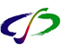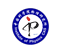引用本文: 汪世清. 谈普朗克质量[J]. 物理, 2002, 31(05).On planck′s mass[J]. PHYSICS, 2002, 31(05).
 Citation: On planck′s mass[J]. PHYSICS, 2002, 31(05).## On planck′s mass

• 摘要: 简要介绍了普朗克于1912年提出的三个基本物理量：普朗克质量、普朗克长度和普朗克时间.它们已被列入1986和1998年基本物理常数表.该文只讨论普朗克质量.假定原子核内存在量子化的核力场，命名其场量子为“引斥子”，其质量推算出恰好等于普朗克质量.由此可用4个耦合常数定量地描述四种相互作用的强度比，从而还可找到一种测定G的新方法.

Abstract: A brief review is presented of three fundamental physical quantities derived by Max Planck in 1912, referred to have as the Planck mass, Planck length and Planck time which had been arranged in the tables of 1986 and 1998 fundamental physical constants. Here we shall only discuss on Planck mass. Suppose that there is a quantized field of the nuclear forces in the nucleus with a quantum unit which we call the attrarepelom with a mass just equal to the Planck mass. Then the intensity ratio of the four mteractions can be described quantitively by their four coupling constants. A new method can then be found to measure the newtonian constant of gravitation, G./下载:  全尺寸图片 幻灯片
• 分享
• 用微信扫码二维码

分享至好友和朋友圈